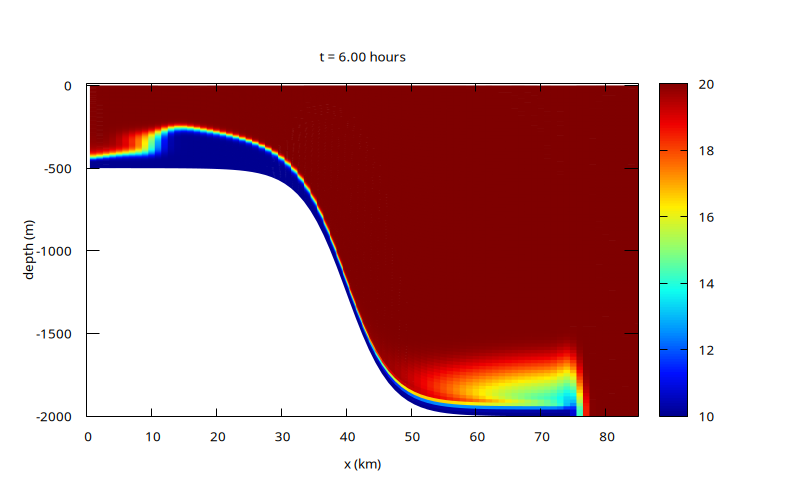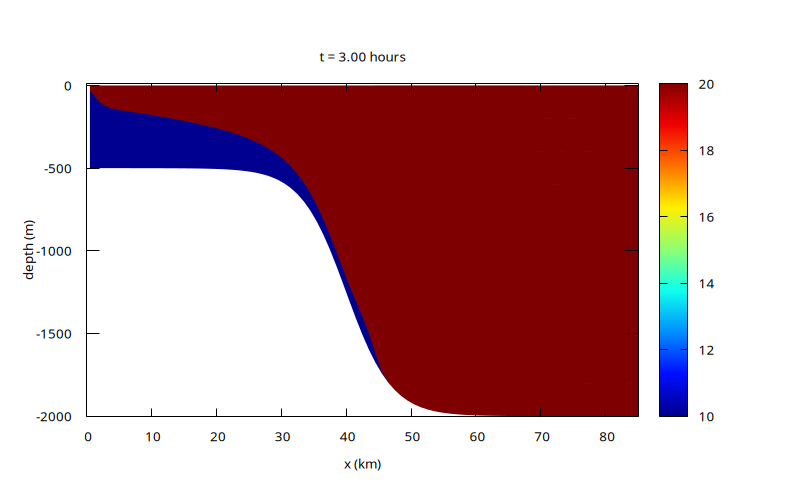# Overflow

This test case was designed by Ilicak et al., 2012, section 4, to investigate the mixing properties of numerical schemes in the case of “overflow” i.e. a denser fluid flowing down a sill or continental slope.

Note that the test case is largely qualitative and is in particular very sensitive to various sources of dissipation, including bottom boundary conditions etc.

The evolution of the “temperature”/density field is illustrated in the sequence below. The cool/dense fluid to the left is released at the top of the slope and flows down. Although no explicit temperature diffusion is prescribed, numerical diffusion leads to the formation of a diffused plume in regions of high shear (the “head” of the flow).

Animation of the temperature field

The figures at t=3 and t=6 hours below agree closely with Figure 4.k and 4.l of Petersen et al, 2015.The amount of mixing can be measured by looking at the evolution of the Reference (or Resting) Potential Energy (RPE) as proposed by Ilicak et al, 2012. In the ideal case without any numerically-induced mixing, the RPE should be conserved.

set xlabel 'Time (hours)'
set ylabel '(RPE - RPE(0))/RPE(0) x 10^5'
hour = 3600.
set xrange [0:12]
plot 'log' u ($1/hour):(-$3/$4*1e7) w l t ''Evolution of the normalised reference potential energy (script) set ylabel 'dRPE/dt (Watts/m^2)' set logscale y L0 = 256e3 plot 'log' u ($1/hour):(abs($3)/$1/L0) w l t ''Evolution of the average rate of change of the reference potential energy (script)

set ylabel 'APE (Joules)'
unset logscale y
plot 'log' every 1 u ($1/hour):($5-$3) w l t ''Evolution of the Available Potential Energy (APE = PE - RPE). (script) set ylabel 'KE (Joules)' plot 'log' every 1 u ($1/hour):6 w l t ''Evolution of the Kinetic Energy. (script)

set ylabel 'Total Energy (Joules)'
unset logscale
plot 'log' every 10 u 1:($5-$3+$6) w l t ''Evolution of the Total Energy KE + APE. (script) ## Non-diffusive interface This is the same setup but using a vertical remapping which preserves the sharp temperature/density interface (i.e. the interface is treated in a Lagrangian manner while the other layers are “Eulerian”). Note that this is different from the two-layers isopycnal run of Petersen et al, 2015 since here 20 layers are used (10 in each “phase”), and so the vertical velocity profile is resolved. Animation of the temperature fieldIn the absence of spurious numerical diffusion, the evolution is much faster and the front propagates much further. As expected the resting potential energy is conserved to within machine accuracy. set xlabel 'Time (hours)' set ylabel '(RPE - RPE(0))/RPE(0) x 10^5' hour = 3600. set xrange [0:12] plot '../overflow-isopycnal/log' u ($1/hour):(-$3/$4*1e7) w l t ''Evolution of the normalised reference potential energy (script)

 [petersen2015] Mark R. Petersen, Douglas W. Jacobsen, Todd D. Ringler, Matthew W. Hecht, and Mathew E. Maltrud. Evaluation of the arbitrary Lagrangian–Eulerian vertical coordinate method in the MPAS-ocean model. Ocean Modelling, 86:93–113, 2015. [ DOI | http ] [ilicak2012] Mehmet Ilicak, Alistair J. Adcroft, Stephen M. Griffies, and Robert W. Hallberg. Spurious dianeutral mixing and the role of momentum closure. Ocean Modelling, 45-46:37–58, 2012. [ DOI | http ]

## Code

We use the 1D (hydrostatic) multilayer solver with a time-implicit discretisation of the (barotropic) free-surface evolution.

#include "grid/multigrid1D.h"
#include "layered/hydro.h"
#include "layered/implicit.h"

We include the Boussineq buoyancy term with a linear “equation of state” linking the temperature T with the relative density variation \Delta\rho(T)/\rho_0.

#define rho0 1000.
#define drho(T) (- (0.2*(T - 5.))/rho0)
#include "layered/dr.h"

The vertical remapping is either uniform (i.e. \sigma-coordinate) or “half-\sigma” in the “isopycnal” case.

#if ISOPYCNAL
# define HALF 1
#endif
#include "layered/remap.h"

This module contains function to compute the (non-trivial) “Resting Potential Energy”.

#include "layered/rpe.h"

We add safety checks on the implicit integration of the free-surface and performance statistics.

#include "layered/check_eta.h"
#include "layered/perfs.h"

The horizontal viscosity is set to the corresponding value in Petersen et al, 2015, Figure 4.k,l. Note however that this has much less influence than in this paper, since most of the effective viscosity comes from the upwind-biased momentum advection scheme.

double nu_H = 1000.; // 1e-2;

int main()
{
size (256e3);
N = 256;

In the “isopycnal” case, we increase the vertical viscosity to try to slow down the gravity current.

#if ISOPYCNAL
nl = 20;
nu = 1e-1;
#else
nl = 100;
nu = 1e-4;
#endif
G = 9.81;
DT = 15.; // Ilicak et al, 2012 use 10 sec

Vertical remapping uses a monotonic limiter to avoid creating new extrema in the temperature field.

  cell_lim = mono_limit;
system ("rm -f plot-*.png"); // fixme: should not be necessary
run();
}

event init (i = 0)
{
foreach() {
double d1 = 500, d2 = 2000, x0 = 40e3, sigma = 7e3;
zb[] = - (d1 + (d2 - d1)/2.*(1. + tanh((x - x0)/sigma)));
foreach_layer() {
#if ISOPYCNAL
T[] = point.l < nl/2 ? 10 : 20;
if (x < 20e3)
h[] = point.l <  nl/2 ? - zb[]/(nl/2) : 1e-2;
else
h[] = point.l >= nl/2 ? - zb[]/(nl/2) : 1e-2;
#else
h[] = - zb[]/nl;
T[] = x < 20e3 ? 10 : 20;
#endif
}
}
}

The bottom boundary slip length corresponding to quadratic bottom friction is given by \displaystyle \lambda_b = \nu\frac{h_0}{C_f|\mathbf{u}|} with C_f the (dimensionless) quadratic bottom friction coefficient.

scalar lambda_q[];

event viscous_term (i++)
{
double Cf = 1e-2;
lambda_b = lambda_q;
foreach() {
double au = norm(u);
lambda_q[] = au < 1e-6 ? HUGE : nu*h[]/(Cf*au);
}
}

### Horizontal viscosity

This is a somewhat naive implementation of horizontal viscosity. Note that we do not use the horizontal_diffusion() function since it does not behave well for zero layer thickness.

event viscous_term (i++)
{
scalar d2u[];
foreach_layer() {
foreach()
d2u[] = (u.x + u.x[-1] - 2.*u.x[])/sq(Delta);
foreach()
u.x[] += dt*nu_H*d2u[];
}
boundary ((scalar *){u});
}

### Outputs

event logfile (i += 10)
{
static double rpe0 = 0., rpen = 0., tn = 0.;
double rpe = rho0*RPE();
double PE, KE;
energy (&PE, &KE);
if (i == 0)
rpe0 = rpe;
fprintf (stderr, "%g %g %.12g %.12g %.12g %.12g %.12g %d\n", t, dt,
rpe - rpe0, rpe0, rho0*PE, rho0*KE,
t > tn ? (rpe - rpen)/(t - tn)/L0 : 0.,
#if NH
mgp.i
#else
mgH.i
#endif
);
rpen = rpe, tn = t;
}

void setup (FILE * fp)
{
fprintf (fp,
#if ISOPYCNAL
"set pm3d map corners2color c2\n"
#else
"set pm3d map\n"
#endif
"# jet colormap\n"
"set palette defined ( 0 0 0 0.5647, 0.125 0 0.05882 1, 0.25"
" 0 0.5647 1, 0.375 0.05882 1 0.9333, 0.5 0.5647 1 0.4392, 0.625"
" 1 0.9333 0, 0.75 1 0.4392 0, 0.875 0.9333 0 0, 1 0.498 0 0 )\n"
"unset key\n"
//	   "set cbrange [10:20]\n"
"set xlabel 'x (km)'\n"
"set ylabel 'depth (m)'\n"
"set xrange [0:200]\n"
"set yrange [-2000:10]\n"
);
}

#define hour 3600.

void plot (FILE * fp)
{
fprintf (fp,
"set title 't = %.2f hours'\n"
"sp '-' u ($1/1e3):2:4\n", t/hour); foreach (serial) { double z = zb[]; fprintf (fp, "%g %g %g %g\n", x, z, u.x[], T[]); foreach_layer() { z += h[]; fprintf (fp, "%g %g %g %g\n", x, z, u.x[], T[]); } fprintf (fp, "\n"); } fprintf (fp, "e\n\n"); // fprintf (fp, "pause 1\n"); fflush (fp); } event gnuplot (t += 600) { static FILE * fp = popen ("gnuplot 2> /dev/null", "w"); if (i == 0) setup (fp); if (getenv ("DISPLAY")) { fprintf (fp, "set term x11\n"); plot (fp); } fprintf (fp, "set term pngcairo font \",10\" size 800,500\n" "set xrange [0:200]\n" "set output 'plot-%04d.png'\n", i); plot (fp); } event figures (t <= 12*hour; t += 3.*hour) { FILE * fp = popen ("gnuplot 2> /dev/null", "w"); setup (fp); fprintf (fp, "set term pngcairo font \",10\" size 800,500\n" "set xrange [0:85]\n" "set output 'T-%g.png'\n", t/hour); plot (fp); } event moviemaker (t = end) { system ("for f in plot-*.png; do convert$f ppm:- && rm -f \$f; done | "
"ppm2mp4 movie.mp4");
}

This optionally displays the diagnostic corresponding to check_eta.h above.

#if 0
#include "deta.h"
#endif